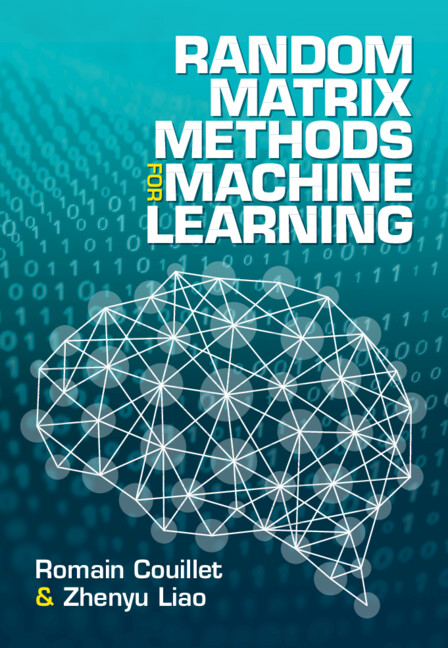# Book### Random Matrix Methods for Machine Learning

Romain Couillet and Zhenyu Liao, Cambridge University Press, 2022.

#### Online Ordering:

If you purchase on the CUP webpage above, a 20% discount flyer is available here.

A pre-production version of the book is available here, with exercise solutions available here. MATLAB and Python codes to reproduce the figures in the book are publicly available in this repository.

Disclaimer: The pre-publication version is free to view and download for personal use only, and is not for redistribution, re-sale or use in derivative works.

## Preface

Numerous and large-dimensional data is now a default setting in modern machine learning (ML). Standard ML algorithms, starting with kernel methods such as support vector machines and graph-based methods like the PageRank algorithm, were however initially designed out of small-dimensional intuitions and tend to misbehave, if not completely collapse, when dealing with real-world large datasets. Random matrix theory has recently developed a broad spectrum of tools to help understand this new “curse of dimensionality,” to help repair or completely recreate the suboptimal algorithms, and most importantly, to provide new intuitions to deal with modern data mining. This book primarily aims to deliver these intuitions, by providing a digest of the recent theoretical and applied breakthroughs of random matrix theory into ML. Targeting a broad audience, spanning from undergraduate students interested in statistical learning to artificial intelligence engineers and researchers alike, the mathematical prerequisites to the book are minimal (basics of probability theory, linear algebra, and real and complex analyses are sufficient): As opposed to introductory books in the mathematical literature of random matrix theory and large-dimensional statistics, the theoretical focus here is restricted to the essential requirements to ML applications. These applications range from detection, statistical inference, and estimation to graph- and kernel-based supervised, semisupervised, and unsupervised classification, as well as neural networks: For these, a precise theoretical prediction of the algorithm performance (often inaccessible when not resorting to a random matrix analysis), large-dimensional insights, methods of improvement, along with a fundamental justification of the wide-scope applicability of the methods to real data, are provided. Most methods, algorithms, and figures proposed in the book are coded in MATLAB and Python and made available to the readers (https://github.com/Zhenyu-LIAO/RMT4ML). The book also contains a series of exercises of two types: short exercises with corrections available online to familiarize the reader with the basic theoretical notions and tools in random matrix analysis, as well as long guided exercises to apply these tools to further concrete ML applications.

• Page 172, Section 3.1.1: “one must choose a threshold $f(\alpha)$ for $T_p$ such that $\mathbb{P} (T_p \geq f(\alpha)) = r$”.
• Page 310, Section 5.1.1: in the equation after “for which the numerator term of $\bar E_{\rm test}$ is now”, the first $\frac1{\hat n}$ should be $\frac1{\hat n} {\rm \tr} (\bar K_{\hat X \hat X})$.